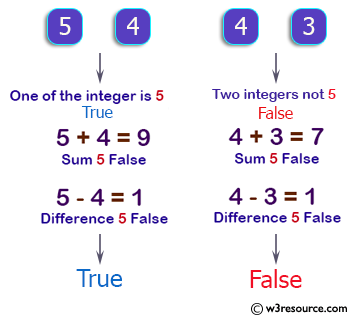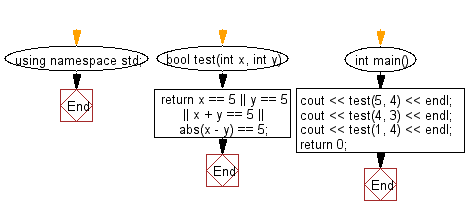﻿ C++ : Check if either one is 5 or difference or sum is 5# C++ Exercises: Accept two integers and return true if either one is 5 or their sum or difference is 5

## C++ Basic Algorithm: Exercise-38 with Solution

Write a C++ program that accept two integers and return true if either one is 5 or their sum or difference is 5.

Sample Solution:

C++ Code :

``````#include <iostream>

using namespace std;

bool test(int x, int y)
{
return x == 5 || y == 5 || x + y == 5 || abs(x - y) == 5;
}

int main()
{
cout << test(5, 4) << endl;
cout << test(4, 3) << endl;
cout << test(1, 4) << endl;
return 0;
}
``````

Sample Output:

```1
0
1
```

Pictorial Presentation:Flowchart:C++ Code Editor: